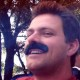## Writing Efficient UDFs Part 12: Getting Used Range Fast using Application Events and a Cache

In the previous post I suggested that one good way to speed up retrieval of the Used Range last row would be to use a Cache and the AfterCalculate Application event.

I have now tested this approach and it works well: here is the code for the demo function GetUsedRows3:

```
Option Explicit
'
' create module level array for cache
'
Dim UsedRows(1 To 1000, 1 To 2) As Variant
Public Function GetUsedRows3(theRng As Range)
' store & retrieve used range rows if Excel 2007 & later
Dim strBookSheet As String
Dim j As Long
Dim nFilled As Long
Dim nRows As Long
' create label for this workbook & sheet
strBookSheet = Application.Caller.Parent.Parent.Name & "_" & Application.Caller.Parent.Name
If Val(Application.Version) >= 12 Then
' look in cache
For j = LBound(UsedRows) To UBound(UsedRows)
If Len(UsedRows(j, 1)) > 0 Then
nFilled = nFilled + 1
If UsedRows(j, 1) = strBookSheet Then
' found
GetUsedRows3 = UsedRows(j, 2)
Exit Function
End If
Else
' exit loop at first empty row
Exit For
End If
Next j
End If
' find used rows
nRows = theRng.Parent.UsedRange.Rows.Count
'
If Val(Application.Version) >= 12 Then
' store in cache
nFilled = nFilled + 1
If nFilled <= UBound(UsedRows) Then
UsedRows(nFilled, 1) = strBookSheet
UsedRows(nFilled, 2) = nRows
End If
End If
'
GetUsedRows3 = nRows
End Function
Sub ClearCache()
'
' empty the first row of the used-range cache
'
UsedRows(1, 1) = ""
End Sub

```

Note: there is no error handling in this code!

Start by defining a module level array (UsedRows) with 1000 rows and 2 columns. Each row will hold a key in column 1 (book name and sheet name) and the number of rows in the used range for that sheet in that book in column 2. I have assumed that we will only cache the first 1000 worksheets containing these UDFs!
The key or label is created by concatenating the name of the parent of the calling cell (which is the worksheet) to the name of the parent of the parent of the calling cell (which is the workbook containing the sheet).
Then loop down the UsedRows array looking for the key, but exit the loop at the first empty row.

If the key is found, retrieve the number of rows in the used range from column 2, return it as the result of the function and exit the function.

Otherwise find the number of rows in the used range,  store it in the next row of the UsedRange cache and return it as the result of the function.

### Only for Excel 2007 or later

You can see that the function only operates the cache for Excel 2007 and later versions. There are two reasons for this:

• Excel 2003 and earlier have a maximum of 64K rows so finding the used range is relatively fast anyway.
• Only Excel 2007 and later have the AfterCalculate event which will be used to empty the cache after each calculate.

We need to empty the cache after each calculate because the user might alter the used range and so the safe thing to do is to recreate the cache at each calculation.
AfterCalculate is an Application Level event which is triggered after completion of a a calculation and associated queries and refreshes. (A BeforeCalculate event would be even more useful but does not exist!)

### Using the AfterCalculate Application Event.

Chip Pearson has an excellent page on Application Events. I always consult it when I need application events because I can never remember exactly how to do it!

First I added a Class Module called AppEvents with code like this:

```
Option Explicit
Private WithEvents App As Application
Private Sub Class_Initialize()
Set App = Application
End Sub
Private Sub App_AfterCalculate()
ClearCache
End Sub

```

Then I added some code to the ThisWorkbook module:

```
Option Explicit
Private XLAppEvents As AppEvents
Private Sub Workbook_Open()
Set XLAppEvents = New AppEvents
End Sub

```

This sets up the hooks that are needed for Application level events. Quite a lot of code just to run the ClearCache sub after each calculation!
ClearCache just empties the first key in the Cache so that the find loop in GetUsedRows3 exits straight away.

This code is ignored in Excel 2003 and earlier: since the AfterCalculate event does not exist it never gets called but still compiles OK.

### Performance of GetUsedRows3

For 640K rows of data 1000 calls to GetUsedRows3 takes 66 milliseconds. The original CountUsedRows function took 33 seconds.
Thats a speedup factor of 500!

This entry was posted in Calculation, UDF, Uncategorized, VBA. Bookmark the permalink.

### 2 Responses to Writing Efficient UDFs Part 12: Getting Used Range Fast using Application Events and a Cache

1.Govert says:

Hi Charles – I found this useful post (again) today – thank you!
I’m curious – for your implementation of this technique with C in the XLL, do you have a way of finding the used range with XLM macro calls, or do you make the COM calls from inside the UDF there too?
-Govert

2.fastexcel says:

Hi Govert, in the XLL a UDF looks in the cache first then if there is nothing cached it makes a locked COM call only if the UDF is executing on the main thread. If the UDF is not on the main thread and there is nothing in the cache then it behaves as if the used range is all the rows (so as to fail safe).